0

# 图嵌入概述：节点、边和图嵌入方法及Python实现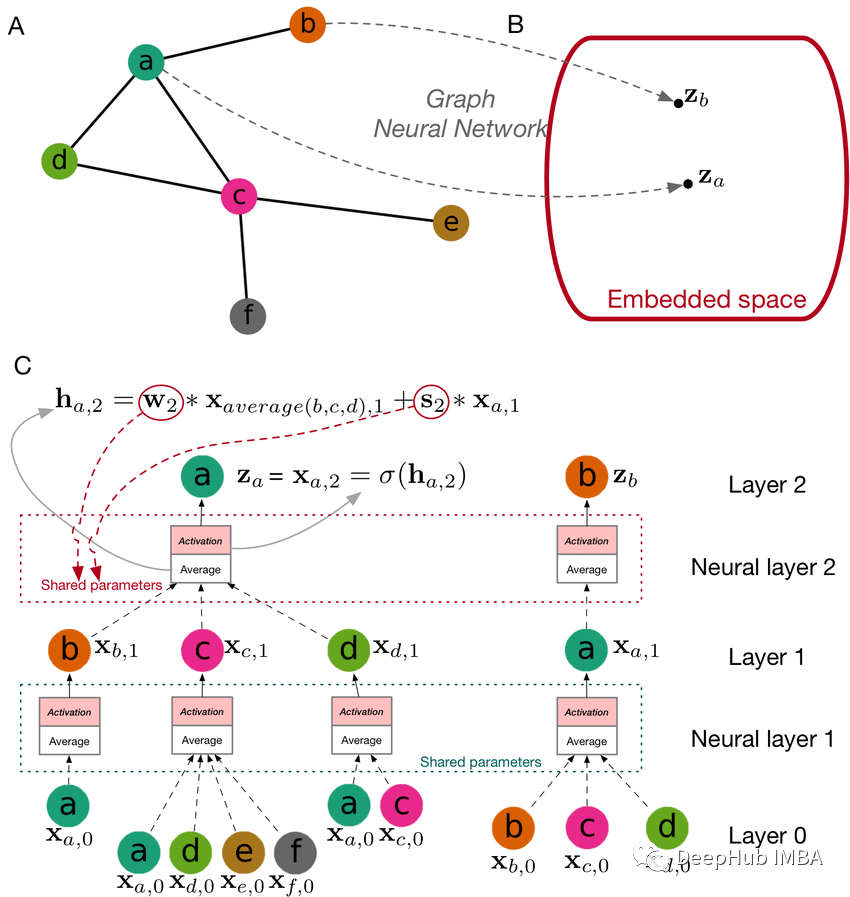## 图嵌入的类型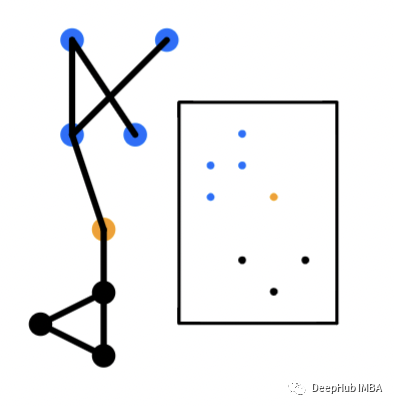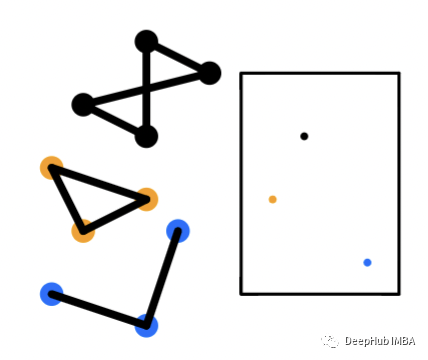## Python实现

`````` Python=3.9
networkx>=2.5
pandas>=1.2.4
numpy>=1.20.1
node2vec>=0.4.4
karateclub>=1.3.3
matplotlib>=3.3.4
``````

`````` import random
import networkx as nx
import matplotlib.pyplot as plt

from node2vec import Node2Vec
from karateclub import Graph2Vec

plt.style.use("seaborn")

# generate barbell network
G = nx.barbell_graph(
m1 = 13,
m2 = 7
)

# node embeddings
def run_n2v(G, dimensions=64, walk_length=80, num_walks=10, p=1, q=1, window=10):
"""
Given a graph G, this method will run the Node2Vec algorithm trained with the
appropriate parameters passed in.

Args:
G (Graph) : The network you want to run node2vec on

Returns:
This method will return a model

Example:
G = np.barbell_graph(m1=5, m2=3)
mdl = run_n2v(G)
"""

mdl = Node2Vec(
G,
dimensions=dimensions,
walk_length=walk_length,
num_walks=num_walks,
p=p,
q=q
)
mdl = mdl.fit(window=window)
return mdl

mdl = run_n2v(G)

# visualize node embeddings
x_coord = [mdl.wv.get_vector(str(x)) for x in G.nodes()]
y_coord = [mdl.wv.get_vector(str(x)) for x in G.nodes()]

plt.clf()
plt.scatter(x_coord, y_coord)
plt.xlabel("Dimension 1")
plt.ylabel("Dimension 2")
plt.title("2 Dimensional Representation of Node2Vec Algorithm on Barbell Network")
plt.show()
``````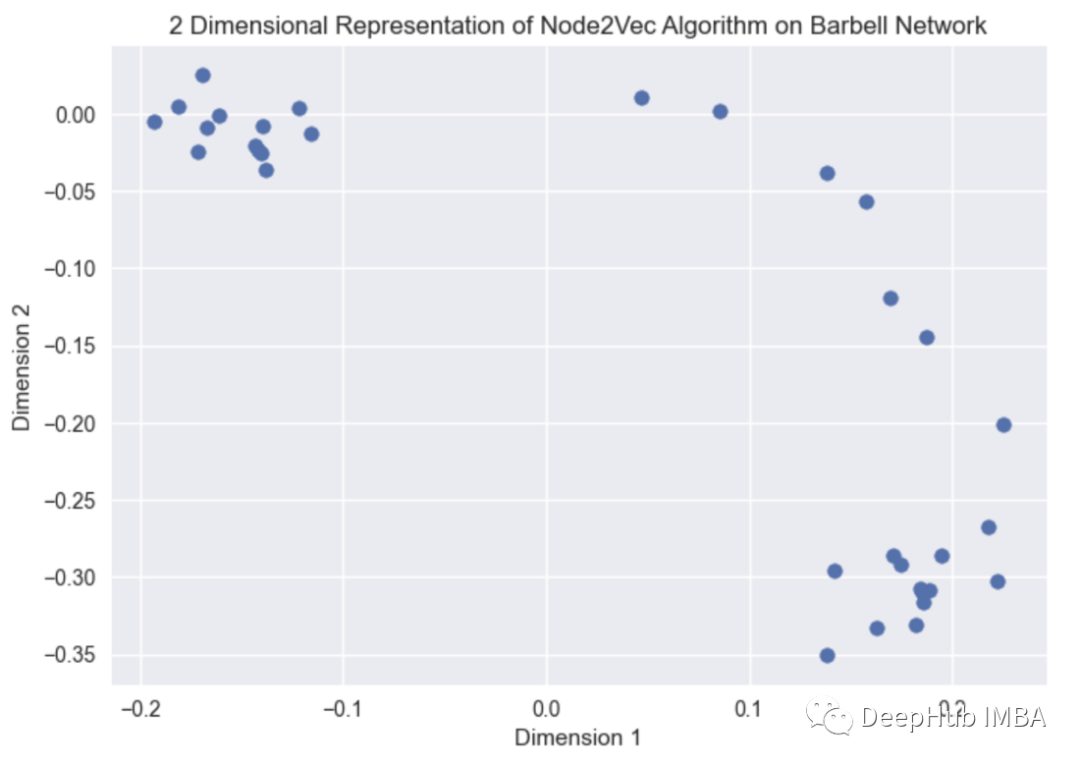`````` edges_embs = HadamardEmbedder(
keyed_vectors=mdl.wv
)

# visualize embeddings
coordinates = [
edges_embs[(str(x), str(x))] for x in G.edges()
]

plt.clf()
plt.scatter(coordinates, coordinates)
plt.xlabel("Dimension 1")
plt.ylabel("Dimension 2")
plt.title("2 Dimensional Representation of Edge Embeddings on Barbell Network")
plt.show()
``````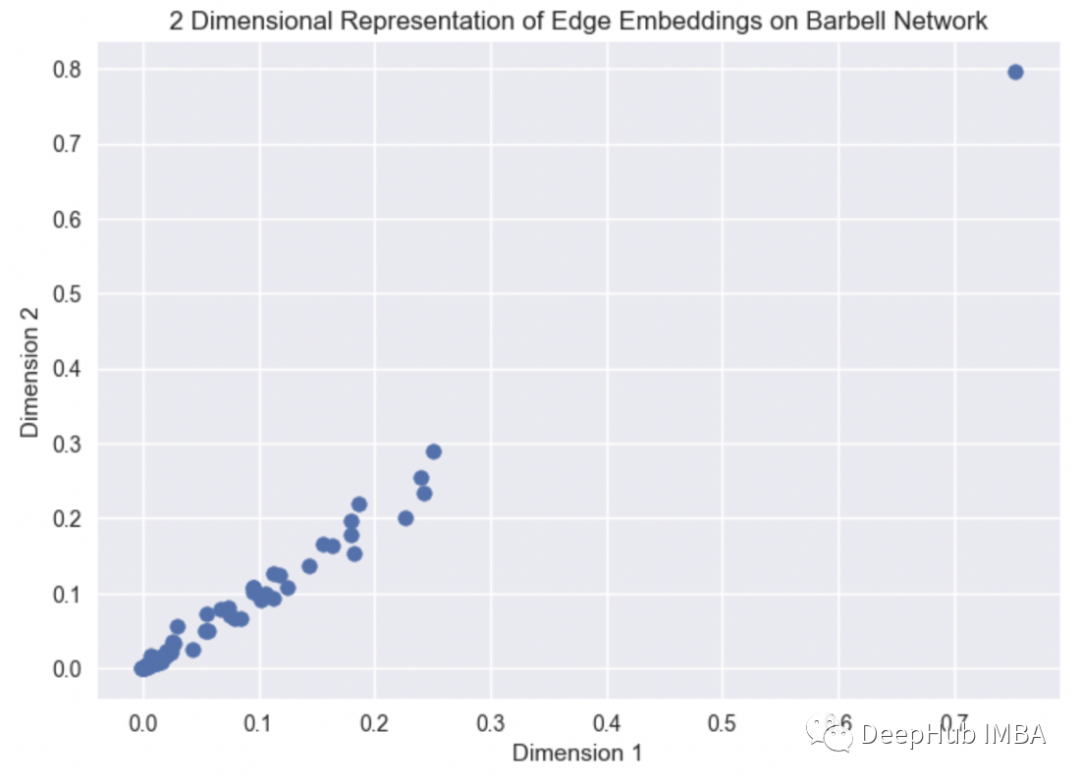`````` n_graphs = 10
Graphs = [
nx.fast_gnp_random_graph(
n = random.randint(5,15),
p = random.uniform(0,1)
) for x in range(n_graphs)
]

g_mdl = Graph2Vec(dimensions=2)
g_mdl.fit(Graphs)
g_emb = g_mdl.get_embedding()

x_coord = [vec for vec in g_emb]
y_coord = [vec for vec in g_emb]

plt.clf()
plt.scatter(x_coord, y_coord)
plt.xlabel("Dimension 1")
plt.ylabel("Dimension 2")
plt.title("2 Dimensional Representation of Graph Embeddings on Randomly Generated Networks")
plt.show()
``````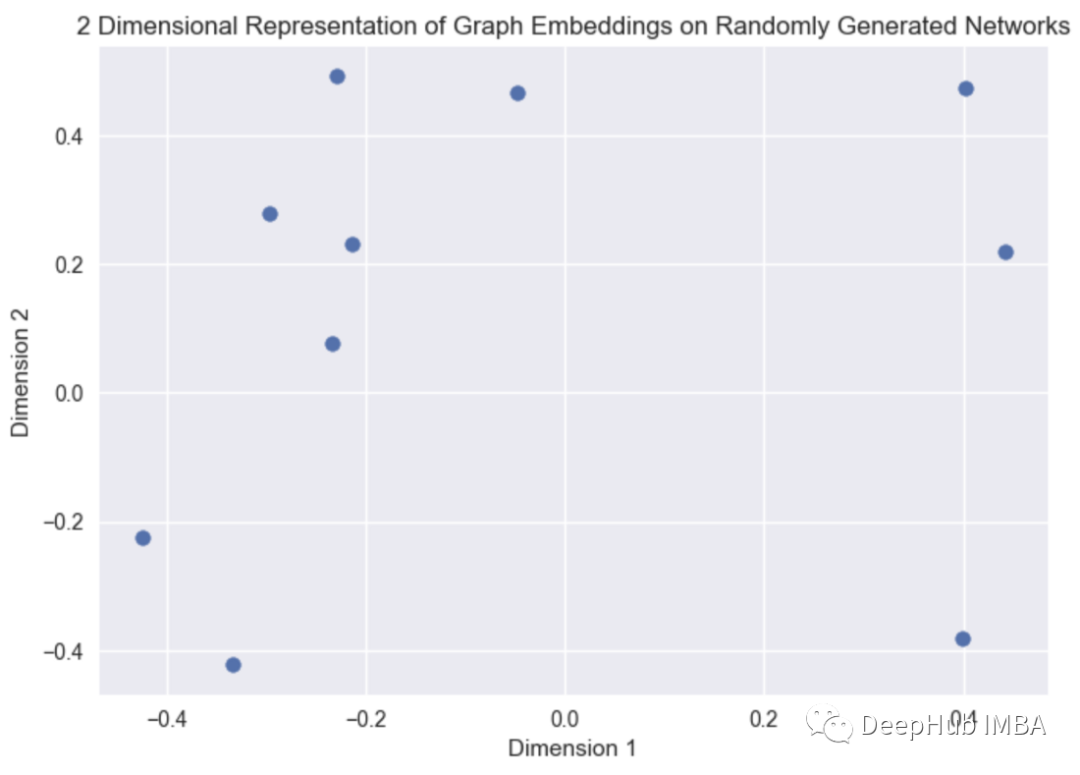## 总结

https://github.com/vatsal220/medium_articles/blob/main/graph_embeddings/graph_emb.ipynb

## 引用

### “图嵌入概述：节点、边和图嵌入方法及Python实现”的评论:

##### 关于作者##### Deephub